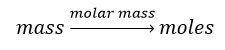# Problem: A deep-sea diver uses a gas cylinder with a volume of 10.0 L and a content of 51.3 g of O2 and 32.7 g of He.Calculate the partial pressure of each gas and the total pressure if the temperature of the gas is 19 oC.

###### FREE Expert Solution

First, calculate the number of moles of O2 and He present in the tank:Molar mass O2 = 16 g/mol x 2 = 32 g/mol
Molar mass He = 4.0 g/mol

96% (198 ratings)###### Problem Details

A deep-sea diver uses a gas cylinder with a volume of 10.0 L and a content of 51.3 g of O2 and 32.7 g of He.

Calculate the partial pressure of each gas and the total pressure if the temperature of the gas is 19 oC.